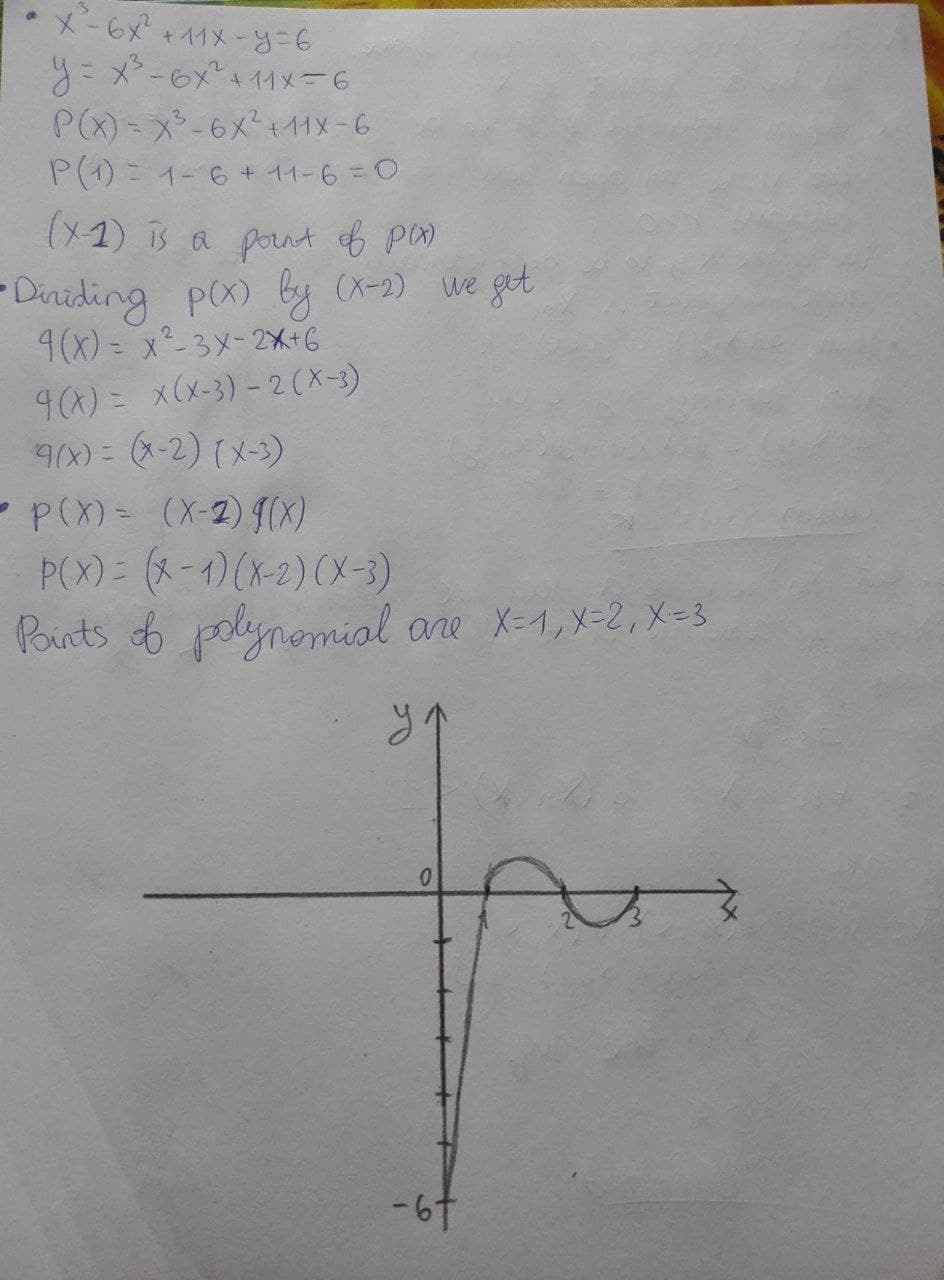# Trace the curve of x^3 - 6x^2 +11x - y = 6zi2lalZ 2021-01-31 Answered
Trace the curve of ${x}^{3}-6{x}^{2}+11x-y=6$
You can still ask an expert for help

• Questions are typically answered in as fast as 30 minutes

Solve your problem for the price of one coffee

• Math expert for every subject
• Pay only if we can solve itAnonym
${x}^{3}-6{x}^{2}+11x-y=6$
$y={x}^{3}-6{x}^{2}+11x-6$
$p\left(x\right)={x}^{3}-6{x}^{2}+11x-6$
$p\left(1\right)=1-6+11-6=0$
(x-1) is a point of p(x)
Dividing p(x) by (x-2) we get
$q\left(x\right)={x}^{2}-3x-21x+6$
$q\left(x\right)=x\left(x-3\right)-2\left(x-3\right)$
$q\left(x\right)=\left(x-2\right)\left(x-3\right)$
$p\left(x\right)=\left(x-1\right)q\left(x\right)$
$p\left(x\right)=\left(x-1\right)\left(x-2\right)\left(x-3\right)$
Point of polynomial are x=1,2,3